# Neural Network for Digital Predistortion Design - Offline Training

This example shows how to use a neural network to apply digital predistortion (DPD) to offset the effects of nonlinearities in a power amplifier (PA). The example focuses on offline training of the neural network-based DPD (NN-DPD). In this example, you

• Generate OFDM signals.

• Send these signals through an actual PA and measure the output.

• Train an NN-DPD.

• Predistort the OFDM signal with the NN-DPD, send this distorted signal through the actual PA, and measure the output to evaluate the effectiveness of the NN-DPD.

• Compare the results to memory polynomial DPD.

### Introduction

Nonlinear behavior in PAs result in severe signal distortions and cause challenges for error-free reception of the high-frequency and high-bandwidth signals commonly transmitted in 5G NR . DPD of the transmitted signal is a technique used to compensate for PA nonlinearities that distort the signal. Typically, the PA nonlinear behavior is characterized in advance and DPD applies an inverse predistortion using some form of memory polynomials . For instance, see the Digital Predistortion to Compensate for Power Amplifier Nonlinearities example. Experimentation with neural network-based DPD techniques shows promising results that offer better performance than the traditional memory polynomial DPD   .

This diagram shows the offline training workflow. First, train an NN-DPD by using the input and output signals of the PA. Then, use the trained NN-DPD.

The upper path shows the neural network training workflow. During training, measure the input to the PA, $u$, and the output of the PA, $x$. To train the neural network as the inverse of the PA and use it for DPD, use $x$ as the input signal and $u$ as the target signal. This architecture is also called indirect learning .

The lower path shows the deployed workflow with the trained NN-DPD inserted before the PA. In this configuration, the NN-DPD inputs the oversampled signal $u$ and output, $y$, as the input to the PA. The PA output $z$ is the linearized signal.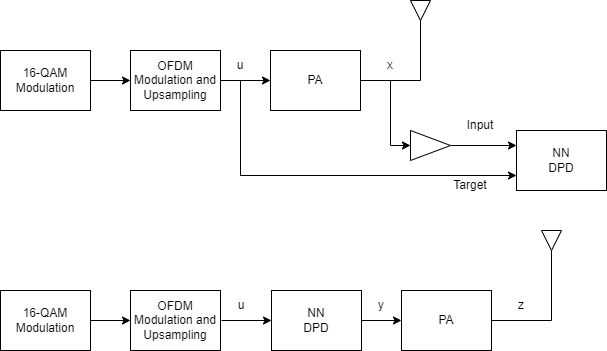### NN-DPD Structure

Design an augmented real-valued time-delay neural network (ARVTDNN) as described in . ARVTDNN has multiple fully connected layers and an augmented input.

The memory polynomial model has been commonly applied in the behavioral modeling and predistortion of PAs with memory effects. This equation shows the PA memory polynomial.

`$\mathit{x}\left(\mathit{n}\right)=\mathit{f}\left(\mathit{u}\left(\mathit{n}\right)\right)=\sum _{\mathit{m}=0}^{\mathit{M}-1}\sum _{\mathit{k}=0}^{\mathit{K}-1}{\mathit{c}}_{\mathit{m}}\mathit{u}\left(\mathit{n}-\mathit{m}\right){|\mathit{u}\left(\mathit{n}-\mathit{m}\right)|}^{\mathit{k}}$`

The output is a function of the delayed versions of the input signal, $u\left(n\right)$, and also powers of the amplitudes of $u\left(n\right)$ and its delayed versions.

Since a neural network can approximate any function provided that it has enough layers and neurons per layer, you can input $u\left(n\right)$ to the neural network and approximate $f\left(u\left(n\right)\right)$. The neural network can input $u\left(n-m\right)$ and $|u\left(n-m\right){|}^{k}$ to decrease the required complexity.

The NN-DPD has multiple fully connected layers. The input layer inputs the in-phase and quadrature components (${\mathit{I}}_{\mathrm{in}}$/${\mathit{Q}}_{\mathrm{in}}$) of the complex baseband samples. The ${\mathit{I}}_{\mathrm{in}}$/${\mathit{Q}}_{\mathrm{in}}$ samples and $m$ delayed versions are used as part of the input to account for the memory in the PA model. Also, the amplitudes of the ${\mathit{I}}_{\mathrm{in}}$/${\mathit{Q}}_{\mathrm{in}}$ samples up to the ${k}^{th}$ power are fed as input to account for the nonlinearity of the PA.During training,

`$\begin{array}{l}{I}_{in}\left(n\right)=\mathrm{\Re }\left(x\left(n\right)\right)\\ {Q}_{in}\left(n\right)=\mathrm{\Im }\left(x\left(n\right)\right)\\ {I}_{out}\left(n\right)=\mathrm{\Re }\left(u\left(n\right)\right)\\ {Q}_{out}\left(n\right)=\mathrm{\Im }\left(u\left(n\right)\right),\end{array}$`

while during deployment (inference),

`$\begin{array}{l}{I}_{in}\left(n\right)=\mathrm{\Re }\left(u\left(n\right)\right)\\ {Q}_{in}\left(n\right)=\mathrm{\Im }\left(u\left(n\right)\right)\\ {I}_{out}\left(n\right)=\mathrm{\Re }\left(y\left(n\right)\right)\\ {Q}_{out}\left(n\right)=\mathrm{\Im }\left(y\left(n\right)\right),\end{array}$`

where $\mathrm{\Re }$ and $\mathrm{\Im }$ are the real and imaginary part operators, respectively.

### Generate Training Data

Generate training, validation, and test data. Use the training and validation data to train the NN-DPD. Use the test data to evaluate the NN-DPD performance.

#### Choose Data Source and Bandwidth

Choose the data source for the system. This example uses an NXP Airfast LDMOS Doherty PA, which is connected to a local NI VST, as described in the Power Amplifier Characterization example. If you do not have access to a PA, run the example with saved data.

`dataSource =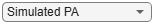"Saved Data";`

#### Generate Oversampled OFDM Signals

Generate OFDM-based signals to excite the PA. This example uses a 5G-like OFDM waveform. Choose the bandwidth of the signal. Choosing a larger bandwidth signal causes the PA to introduce more nonlinear distortion and yields greater benefit from the addition of DPD. Generate six OFDM symbols, where each subcarrier carries a 16-QAM symbol, using the `ofdmmod` and `qammod` function. Save the 16-QAM symbols as a reference to calculate the EVM performance. To capture effects of higher order nonlinearities, the example oversamples the PA input by a factor of 7.

```if strcmp(dataSource,"NI VST") bw =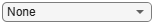100e6; % Hz numOFDMSym = 6; % 6 OFDM symbols per frame M = 16; % Each OFDM subcarrier contains a 16-QAM symbol ofdmParams = helperOFDMParameters(bw); ofdmParams.osr = 7; % oversample rate for PA input [paInputTrain,qamRefSymTrain,sr] = ... helperNNDPDGenerateOFDM(ofdmParams,numOFDMSym, M);```

Pass the signal through the PA and measure the output signal. Lower target input power values may cause less distortion. For this setup, when the signal is predistorted, 5 dBm is the maximum value the NI PXIe-4139 SMU described in the Power Amplifier Characterization example can support without saturation.

``` targetInputPower =5; % dBm paOutputTrain = helperNNDPDPAMeasure(paInputTrain,targetInputPower,sr);```

Repeat the same procedure to generate validation and test data.

``` % Generate validation data [paInputVal,qamRefSymVal] = ... helperNNDPDGenerateOFDM(ofdmParams,numOFDMSym,M); paOutputVal = helperNNDPDPAMeasure(paInputVal,targetInputPower,sr); % Generate test data [paInputTest,qamRefSymTest] = ... helperNNDPDGenerateOFDM(ofdmParams,numOFDMSym,M); paOutputTest = helperNNDPDPAMeasure(paInputTest,targetInputPower,sr); if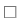false % Select true to save data for saved data workflow save savedData bw numOFDMSym M ofdmParams sr targetInputPower ... qamRefSymTrain paInputTrain paOutputTrain qamRefSymVal ... paInputVal paOutputVal qamRefSymTest paInputTest paOutputTest %#ok<UNRCH> end elseif strcmp(dataSource,"Saved Data") helperNNDPDDownloadData() load("savedDataNIVST100MHz"); end```
```Starting download of data files from: https://www.mathworks.com/supportfiles/spc/NNDPD/NNDPD_deeplearning_uploads.zip Download complete. Extracting files. Extract complete. ```

 and  describe the benefit of normalizing the input signal to avoid the gradient explosion problem and ensure that the neural network converges to a better solution. Normalization requires obtaining a unity standard deviation and zero mean. For this example, the communication signals already have zero mean, so normalize only the standard deviation. Later, you need to denormalize the NN-DPD output values by using the same scaling factor.

```scalingFactor = 1/std(paInputTrain); paInputTrainNorm = paInputTrain*scalingFactor; paOutputTrainNorm = paOutputTrain*scalingFactor; paInputValNorm = paInputVal*scalingFactor; paOutputValNorm = paOutputVal*scalingFactor; paInputTestNorm = paInputTest*scalingFactor; paOutputTestNorm = paOutputTest*scalingFactor;```

### Implement and Train NN-DPD

Before training the neural network DPD, select the memory depth and degree of nonlinearity. For purposes of comparison, specify a memory depth of 5 and a nonlinear polynomial degree of 5, as in the Power Amplifier Characterization example, and will be used to compare performance. Then implement the network described in Neural Network DPD Structure section.

```memDepth = 5; % Memory depth of the DPD (or PA model) nonlinearDegree = 5; % Nonlinear polynomial degree inputLayerDim = 2*memDepth+(nonlinearDegree-1)*memDepth; numNeuronsPerLayer = 40; lgraph = [... featureInputLayer(inputLayerDim,'Name','input') fullyConnectedLayer(numNeuronsPerLayer,'Name','linear1') leakyReluLayer(0.01,'Name','leakyRelu1') fullyConnectedLayer(numNeuronsPerLayer,'Name','linear2') leakyReluLayer(0.01,'Name','leakyRelu2') fullyConnectedLayer(numNeuronsPerLayer,'Name','linear3') leakyReluLayer(0.01,'Name','leakyRelu3') fullyConnectedLayer(2,'Name','linearOutput') regressionLayer('Name','output')];```

#### Prepare Input Data Vector

Create the input vector. During training and validation, use the PA output as NN-DPD input and the PA input as the NN-DPD output.

```% Create input layer arrays for each time step as a matrix for training, % validation and test signals. inputProc = helperNNDPDInputLayer(memDepth,nonlinearDegree); inputTrainMtx = process(inputProc,paOutputTrainNorm); inputTrainMtx = inputTrainMtx(memDepth+1:end,:); reset(inputProc) inputValMtx = process(inputProc,paOutputValNorm); inputValMtx = inputValMtx(memDepth+1:end,:); reset(inputProc) inputTestMtx = process(inputProc,paInputTestNorm); inputTestMtx = inputTestMtx(memDepth+1:end,:); % Create outputs as two element [I Q] vectors for each time step outputTrainMtx = [real(paInputTrainNorm(memDepth+1:end,:)), ... imag(paInputTrainNorm(memDepth+1:end,:))]; outputValMtx = [real(paInputValNorm(memDepth+1:end,:)), ... imag(paInputValNorm(memDepth+1:end,:))]; outputTestMtx = [real(paOutputTestNorm(memDepth+1:end,:)), ... imag(paOutputTestNorm(memDepth+1:end,:))];```

#### Train Neural Network

Train the neural network offline using the `trainNetwork` (Deep Learning Toolbox) function. First, define the training options using the `trainingOptions` (Deep Learning Toolbox) function and set hyperparameters. Use the Adam optimizer with a mini-batch size of 256. The initial learning rate is 1e-4 and decreases by a factor of 0.95 every two epochs. Evaluate the training performance using validation every 1000 iterations. If the validation accuracy does not increase for five validations, stop training. Use Experiment Manager (Deep Learning Toolbox) to optimize hyperparameters.

```maxEpochs = 200; miniBatchSize = 256; options = trainingOptions('adam', ... MaxEpochs=maxEpochs, ... MiniBatchSize=miniBatchSize, ... InitialLearnRate=1e-4, ... LearnRateDropFactor=0.95, ... LearnRateDropPeriod=2, ... LearnRateSchedule='piecewise', ... Shuffle='every-epoch', ... OutputNetwork='best-validation-loss', ... ValidationData={inputValMtx,outputValMtx}, ... ValidationFrequency=1000, ... ValidationPatience=5, ... Plots='training-progress', ... Verbose=false);```

When running the example, you have the option of using a pretrained network by setting the `trainNow` variable to `false`. Training is desirable to match the network to your simulation configuration. If using a different PA, signal bandwidth, or target input power level, retrain the network. Training the neural network on an Nvidia® Titan V GPU takes about 40 minutes.

```trainNow =false; if trainNow netDPD = trainNetwork(inputTrainMtx,outputTrainMtx,lgraph,options); %#ok<UNRCH> iffalse % Select true to save data for saved data workflow save savedNet netDPD end else load('savedNetNIVST100MHz'); end```

The following shows the training process with the given options. Since the root mean squared error (RMSE) does not change much after about 40 epochs, you can reduce the training time to less than 15 minutes by setting the maximum epochs to 40 without losing much performance.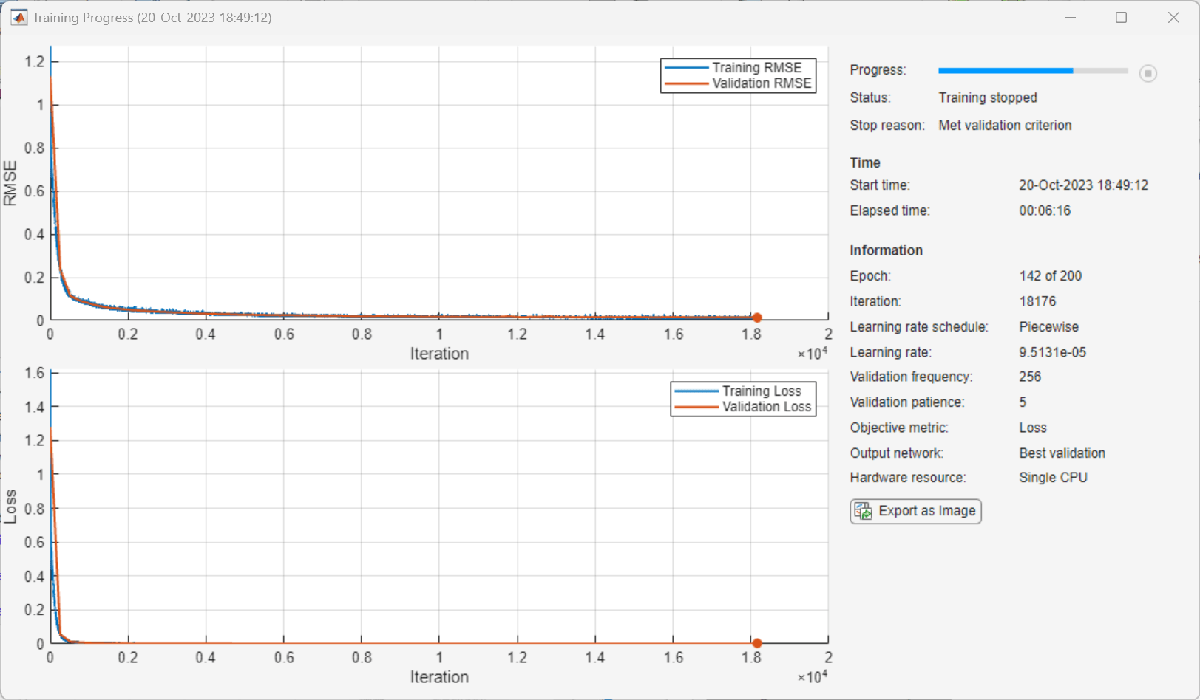### Test NN-DPD

This figure shows how to check the performance of the NN-DPD. To test the NN-DPD, pass the test signal through the NN-DPD and the PA and examine these performance metrics:

• Normalized mean square error (NMSE), measured between the input to the NN-DPD and output of the PA

• Adjacent channel power ratio (ACPR), measured at the output of the PA by using the `comm.ACPR` System object

• Percent RMS error vector magnitude (EVM), measured by comparing the OFDM demodulation output to the 16-QAM modulated symbols by using the `comm.EVM` System object

Perform these tests for both the NN-DPD and also the memory polynomial DPD described in the Digital Predistortion to Compensate for Power Amplifier Nonlinearities example.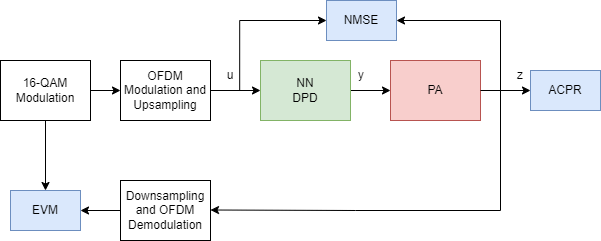```if strcmp(dataSource,"NI VST") % Pass signal through NN-DPD dpdOutNN = predict(netDPD,inputTestMtx); dpdOutNN = [zeros(memDepth,1);... double(complex(dpdOutNN(:,1),dpdOutNN(:,2)))]; dpdOutNN = dpdOutNN/scalingFactor; paOutputNN = helperNNDPDPAMeasure(dpdOutNN,targetInputPower,sr); % Pass signal through memory polynomial DPD dpdOutMP = helperNNDPDMemoryPolynomial(paInputTest,paInputTrain, ... paOutputTrain,nonlinearDegree,memDepth); paOutputMP = helperNNDPDPAMeasure(dpdOutMP,targetInputPower,sr); iffalse % Select true to save data for saved data workflow save savedTestResults paOutputNN dpdOutNN dpdOutMP paOutputMP %#ok<UNRCH> end elseif strcmp(dataSource,"Saved Data") load('savedTestResultsNIVST100MHz'); end % Evaluate performance with NN-DPD acprNNDPD = localACPR(paOutputNN,sr,bw); nmseNNDPD = localNMSE(paInputTest,paOutputNN); evmNNDPD = localEVM(paOutputNN,qamRefSymTest,ofdmParams); % Evaluate the performance without DPD acprNoDPD = localACPR(paOutputTest,sr,bw); nmseNoDPD = localNMSE(paInputTest,paOutputTest); evmNoDPD = localEVM(paOutputTest,qamRefSymTest,ofdmParams); % Evaluate the performance with memory polynomial DPD acprMPDPD = localACPR(paOutputMP,sr,bw); nmseMPDPD = localNMSE(paInputTest,paOutputMP); evmMPDPD = localEVM(paOutputMP,qamRefSymTest,ofdmParams); % Create a table to display results evm = [evmNoDPD;evmMPDPD;evmNNDPD]; acpr = [acprNoDPD;acprMPDPD;acprNNDPD]; nmse = [nmseNoDPD;nmseMPDPD;nmseNNDPD]; disp(table(acpr,nmse,evm, ... 'VariableNames', ... {'ACPR_dB','NMSE_dB','EVM_percent'}, ... 'RowNames', ... {'No DPD','Memory Polynomial DPD','Neural Network DPD'}))```
``` ACPR_dB NMSE_dB EVM_percent _______ _______ ___________ No DPD -28.837 -22.063 5.859 Memory Polynomial DPD -35.547 -29.401 2.3126 Neural Network DPD -38.79 -31.877 1.8642 ```
```sa = helperPACharPlotSpectrum(... [paOutputTest paOutputMP paOutputNN], ... {'No DPD','Memory Polynomial DPD', ... 'Neural Network DPD'}, ... sr,"Modulated",[-130 -50]);```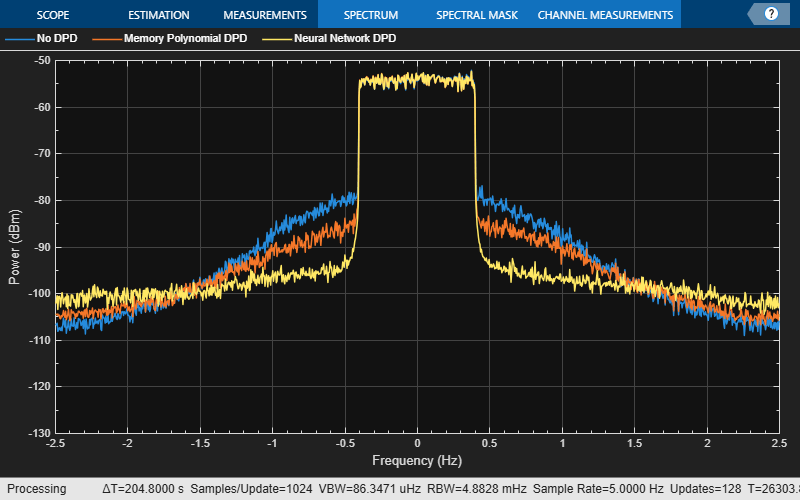As the PA heats, the performance characteristics change. Send bursty signals through the PA repeatedly and plot system performance as a function of time. Each measurement takes about 6 s. Every 600 s, stop for 300 s to allow the PA to cool down. The plot shows that the system performance degrades with repeated use and recovers after the cooldown period. This behavior shows that after some time, the PA characteristics might change and the DPD might not provide the required system performance, such as a maximum EVM value. If the EVM value exceeds the allowed maximum value, the neural network needs to be retrained to adapt to the changing PA characteristics.

```runRepeatedBurstTest =false; if strcmp(dataSource,"NI VST") && runRepeatedBurstTest numMeas = 500; measTime = 6; acprNNDPD = zeros(numMeas,1); nmseNNDPD = zeros(numMeas,1); evmNNDPD = zeros(numMeas,1); [acprLine,nmseLine,evmLine] = initFigure(); tStart = tic; cnt = 1; for p=1:numMeas % Pass signal through NN-DPD dpdOutNN = predict(netDPD,inputTestMtx); dpdOutNN = [zeros(memDepth,1);... double(complex(dpdOutNN(:,1), dpdOutNN(:,2)))]; paInput = dpdOutNN/scalingFactor; % Pass signals through PA paOutputNN = helperNNDPDPAMeasure(paInput, targetInputPower, sr); % Evaluate performance with NN-DPD acprNNDPD(cnt) = localACPR(paOutputNN,sr,bw); nmseNNDPD(cnt) = localNMSE(paInputTest,paOutputNN); evmNNDPD(cnt) = localEVM(paOutputNN,qamRefSymTest,ofdmParams); updateFigure(acprLine,nmseLine,evmLine, ... acprNNDPD(cnt),nmseNNDPD(cnt),evmNNDPD(cnt),tStart); cnt = cnt +1; if mod(p,100) == 0 for q=1:50 pause(measTime) acprNNDPD(cnt) = NaN; nmseNNDPD(cnt) = NaN; evmNNDPD(cnt) = NaN; updateFigure(acprLine,nmseLine,evmLine, ... acprNNDPD(cnt),nmseNNDPD(cnt),evmNNDPD(cnt),tStart); cnt = cnt +1; end end end else load('savedRepeatTestResultsNIVST100MHz'); figure numMeas = length(acprNNDPD); t = (0:numMeas-1)*6; subplot(3,1,1) plot(t,acprNNDPD) grid on title("NN-DPD Performance over Many Bursts") ylabel("ACPR") subplot(3,1,2) plot(t,nmseNNDPD) grid on ylabel("NMSE") subplot(3,1,3) plot(t,evmNNDPD) grid on ylabel("EVM") xlabel('t (s)') end```### Further Exploration

This example demonstrates how to train a NN-DPD by using measured data from a PA. For the given PA, target input power level, and driving signal, the NN-DPD is able to provide better performance than memory polynomial DPD.

You can try changing the number of neurons per layer, number of hidden layers and target input power level and see the effect of these parameters on the NN-DPD performance. You can also try different input signals, such as OFDM signals with different bandwidth. You can also generate standard-specific signals using the Wireless Waveform Generator app.

### Helper Functions

OFDM Signal Generation

Signal Measurement and Input Processing

Performance Evaluation and Comparison

### Local Functions

```function acpr = localACPR(paOutput,sr,bw) %localACPR Adjacent channel power ratio (ACPR) % A = localACPR(X,R,BW) calculates the ACPR value for the input signal X, % for an assumed signal bandwidth of BW. The sampling rate of X is R. acprModel = comm.ACPR(... 'SampleRate',sr, ... 'MainChannelFrequency',0, ... 'MainMeasurementBandwidth',bw, ... 'AdjacentChannelOffset',[-bw bw], ... 'AdjacentMeasurementBandwidth',bw); acpr = acprModel(paOutput); acpr = mean(acpr); end```
```function nmseIndB = localNMSE(input,output) %localNMSE Normalized mean squared error (NMSE) % E = localNMSE(X,Y) calculates the NMSE between X and Y. nmse = sum(abs(input-output).^2) / sum(abs(input).^2); nmseIndB = 10*log10(nmse); end```
```function [rmsEVM,rxQAMSym] = localEVM(paOutput,qamRefSym,ofdmParams) %localEVM Error vector magnitude (EVM) % [E,Y] = localEVM(X,REF,PARAMS) calculates EVM for signal, X, given the % reference signal, REF. X is OFDM modulated based on PARAMS. % Downsample and demodulate waveform = ofdmdemod(paOutput,ofdmParams.fftLength,ofdmParams.cpLength,... ofdmParams.cpLength,[1:ofdmParams.NumGuardBandCarrier/2+1 ... ofdmParams.fftLength-ofdmParams.NumGuardBandCarrier/2+1:ofdmParams.fftLength]',... OversamplingFactor=ofdmParams.osr); rxQAMSym = waveform(:)*ofdmParams.osr; % Compute EVM evm = comm.EVM; rmsEVM = evm(qamRefSym,rxQAMSym); end function [acprLine,nmseLine,evmLine] = initFigure() %initFigure Initialize repeat runs figure figure subplot(3,1,1) acprLine = animatedline; grid on ylabel("ACPR (dB)") title("NN-DPD Performance Over Many Bursts") subplot(3,1,2) nmseLine = animatedline; grid on ylabel("NMSE (dB)") subplot(3,1,3) evmLine = animatedline; grid on ylabel("EVM (%)") xlabel("t (s)") end function updateFigure(acprLine,nmseLine,evmLine,acprNNDPD,nmseNNDPD,evmNNDPD,tStart) %updateFigure Update repeat runs figure addpoints(acprLine,toc(tStart),acprNNDPD) addpoints(nmseLine,toc(tStart),nmseNNDPD) addpoints(evmLine,toc(tStart),evmNNDPD) drawnow limitrate end```

### References

 Tarver, Chance, Liwen Jiang, Aryan Sefidi, and Joseph R. Cavallaro. “Neural Network DPD via Backpropagation through a Neural Network Model of the PA.” In 2019 53rd Asilomar Conference on Signals, Systems, and Computers, 358–62. Pacific Grove, CA, USA: IEEE, 2019. https://doi.org/10.1109/IEEECONF44664.2019.9048910.

 Morgan, Dennis R., Zhengxiang Ma, Jaehyeong Kim, Michael G. Zierdt, and John Pastalan. “A Generalized Memory Polynomial Model for Digital Predistortion of RF Power Amplifiers.” IEEE Transactions on Signal Processing 54, no. 10 (October 2006): 3852–60. https://doi.org/10.1109/TSP.2006.879264.

 Wu, Yibo, Ulf Gustavsson, Alexandre Graell i Amat, and Henk Wymeersch. “Residual Neural Networks for Digital Predistortion.” In GLOBECOM 2020 - 2020 IEEE Global Communications Conference, 01–06. Taipei, Taiwan: IEEE, 2020. https://doi.org/10.1109/GLOBECOM42002.2020.9322327.

 Wang, Dongming, Mohsin Aziz, Mohamed Helaoui, and Fadhel M. Ghannouchi. “Augmented Real-Valued Time-Delay Neural Network for Compensation of Distortions and Impairments in Wireless Transmitters.” IEEE Transactions on Neural Networks and Learning Systems 30, no. 1 (January 2019): 242–54. https://doi.org/10.1109/TNNLS.2018.2838039.

 Sun, Jinlong, Juan Wang, Liang Guo, Jie Yang, and Guan Gui. “Adaptive Deep Learning Aided Digital Predistorter Considering Dynamic Envelope.” IEEE Transactions on Vehicular Technology 69, no. 4 (April 2020): 4487–91. https://doi.org/10.1109/TVT.2020.2974506.

 Sun, Jinlong, Wenjuan Shi, Zhutian Yang, Jie Yang, and Guan Gui. “Behavioral Modeling and Linearization of Wideband RF Power Amplifiers Using BiLSTM Networks for 5G Wireless Systems.” IEEE Transactions on Vehicular Technology 68, no. 11 (November 2019): 10348–56. https://doi.org/10.1109/TVT.2019.2925562.

 Paaso, Henna, and Aarne Mammela. “Comparison of Direct Learning and Indirect Learning Predistortion Architectures.” In 2008 IEEE International Symposium on Wireless Communication Systems, 309–13. Reykjavik: IEEE, 2008. https://doi.org/10.1109/ISWCS.2008.4726067.